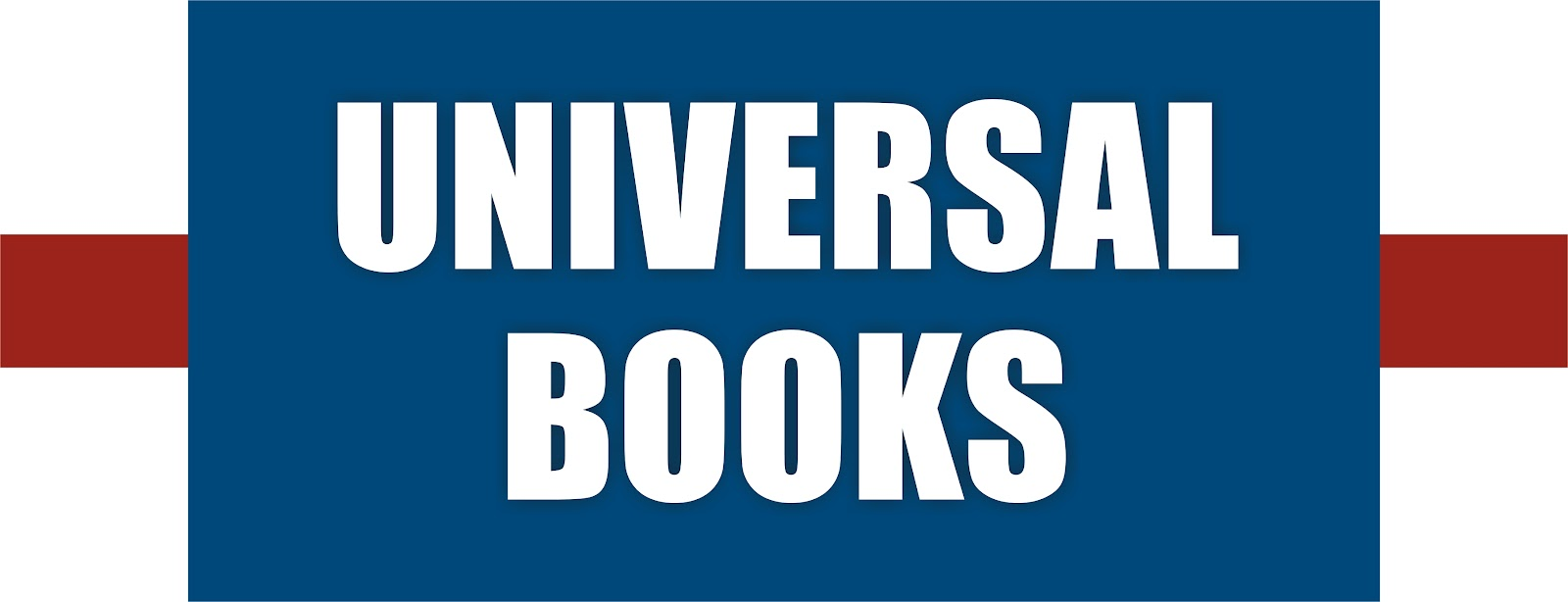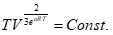PHYSICS NEET

 CHAPTER NAME LOCATION ERROR Chapter 2 : Motion Under Gravity Questions Page 60, Q. 30 Download Questions here:

CHEMISTRY JEE

 CHAPTER NAME LOCATION ERROR Structure of Atom Page 107, Q. 28 Option (d) is correct Classification of Elements and Periodicity in Properties Page 159, Q. 28 None of the options (F

PHYSICS JEE

 Alternating Current Page 1599, Q.100 Question is missing Alternating Current Page 1600, Q.114 Correct answer is option (b) Alternating Current Page 1603, Q.155 No Answer is correct in given option, Correct answer is 31.8 Hz Electron, Photon Photoelectric Effect Page 1670, Q. 25 Solution and answer key is wrong, Correct answer is (d). Ray Optics Page 1890, Q.21 Solution is correct but answer key is wrong, Correct option is (b) Ray Optics Page 1899, Q.73 Solution is correct but answer key is wrong, Correct option is (d) Wave Optics Page 2007, Q.48 Solution is wrong but answer key is correct, Correct option is (b) Wave Optics Page 2016, Q.103 Solution is correct but answer key is wrong, Correct option is (b) Communication Page 1863, Q. 23 Solution is correct but answer key is wrong, Correct option is (c) Thermodynamics Page ix, Q.12 Solution is correct but answer key is wrong, Correct option is (c) Units, Dimensions and Measurement Page 81, Q.34 Solution is correct but answer key is wrong, Correct option is (c) Rotational Motion Page 433, Q. 37 Question is incomplete. Gravitation Page 534, Q.64 Plots (a) & (c) and (b) & (d) are same in Options. Correct option is (c) . Work, Energy, Power and Collision Page 341, Q.107 Option (a) and (c) are same. Correct option is (d). Newton's Laws of Motion Page 262, Q. 30 In all options units are wrong and the correct unit is ms-2 Electrostatics Page 1123, Q.98 In all options units are wrong and the correct unit is μF. Fluids Mechanics Page 675, Q.7 Option (ab) is correct. (c) is not correct. Rotational Motion Page 490, Q.89 correct option is (b) Transmission of Heat Page 852, Q.11 correct option is (a) Transmission of Heat Page 853, Q.18 correct option is (c) Transmission of Heat Page 854, Q.27 correct answer is 9. Transmission of Heat Page 854, Q.28 correct answer is 1/3. Magnetic Effect of Current Page 1423, Q. 94 Solution is correct but answer key is wrong, Correct option is (a) Magnetic Effect of Current Page 1396, Q.57 Solution and answer key is wrong, Correct answer is (c). Simple Harmonic Motion Page 912, Q.31 Solution and answer key is correct.Error in final answer, Correct answer is  T = .30 (approx) Alternating Current Page 1599, Q.100 Question is incomplete in book. Electronics Page 1828, Q. 38 Error in question. Take collector supply voltage as current gain. Electronics Page 1834, Q.39 Error in question. Wave Optics Page 2006, Q.39 Solution is correct but answer key is wrong, Correct option is (d). Wave Optics Page 2016, Q.103 Solution is correct but answer key is wrong, Correct option is (b). Alternating Current Page 1590, Q. 22 Solution and answer key is wrong, Correct answer is (b). Thermodynamics Page 783, Q.21 Solution is correct but answer key is wrong, Correct option is (b). Thermodynamics Page 785, Q.32 It is a controversial Question of KVPY 2016. The correct answer isWork, Energy, Power and Collision Page 338, Q.88 Solution and answer key is wrong, Correct answer is (b). Work, Energy, Power and Collision Page 342, Q.17 Solution and answer key is wrong, Correct answer is (c). Motion in one dimension Page 105, Q.62 Solution and answer key is wrong, Correct answer is (d). Motion in one dimension Page 113, Q.1 Solution and answer key is wrong, Correct answer is (b). Electrostatics Page 1147, Q.73 Solution is incomplete and  answer key is wrong, Correct option is (c). Electrostatics Page 1170, Q.3 Solution is correct but answer key is wrong, Correct option is (b). Current Electricity Page 1290, Q.27 Diagram is missing in this Question. Thermodynamics Page (xx), Q.34 Solution is correct but answer key is wrong. Correct answer is option (c) Newton's Laws of Motion Page 258, Q. 40 Diagram is missing in Question  but answer key is correct, Correct option is (d). Rotational Motion Page 476, Q.35 Solution is wrong  but answer key is correct, Correct option is (a) Simple Harmonic Motion Page 911, Q.14 Solution and answer key is wrong, Correct answer is (c). Rotational Motion Page 428, Q.54 Solution is wrong Rotational Motion Page 447, Q.64 The question is incomplete. Rotational Motion Page 449, Q.33 The correct answer  in options  is missing. The correct answer is given in solution.i.e. 1470 J. Ray Optics Page 1893, Q.28 Answer Key and Solution are wrong. Correct answer is option (b). Units, Dimensions and Measurement Page 50, Q. 50 The given dimension in question and solution is wrong. Units, Dimensions and Measurement Page 51, Q. 60 The given dimension in options are wrong. Magnetism Page 1476, Q. 68 Solution is correct but answer key is wrong. Correct answer is option (a) Magnetic Effect of current Page 1427, Q. 2 Answer Key and Solution is wrong. Correct answer is option (d). Magnetic Effect of Current Page 1441, Q. 129 Solution is wrong